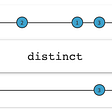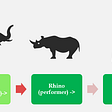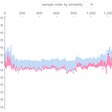# Python Basic

## Related Articles

`print("Hello World")`

# Input and Output

`name = input('Type your name: ')print(name)`

# Data Types

• str (string)
• int (integer)
• float (float)
• bool (boolean)
• list(array ➜ multiple values with multiple datatype)
• tuple(array ➜ multiple values with same datatype)
• dict(dictionary ➜ multiple values with key values)
`# string(text)print("My Name is John")# integer(number)print(899)# float(decimal)print(99.432577834)# boolean(True or False)print(True)print(False)# list(multiple values with multiple datatype)print(['hello', 1, False])# tuple(multiple values with same datatype)print(('orange', 'pink', 'red'))# dict(multiple values with key values)print({'name':'Sophia', 'gender':'female', 'age':20, 'graduated': False, 'favourite_color': ('orange', 'pink', 'red')})`

You can use comments when you want to give some instructions to other programmers. They will not print out.

`# this is comment`

# Variables

`name = "Sophia"graduated = Falseage = 20# different data typesnew_list = ['hello', 1, False]# different data typesnew_tuple = ('orange', 'pink', 'red')# dict data typenew_dict = {'name':'Sophia', 'gender':'female', 'age':20, 'graduated': False, 'favourite_color': ('orange', 'pink', 'red')}print(name)`

Print the value in a list one by one

`new_list = ['hello', 1, False]print(new_list)print(new_list)print(new_list)`

Print the value in a tuple one by one

`new_tuple = ('orange', 'pink', 'red')print(new_tuple)print(new_tuple)print(new_tuple)`

Print the values in a dictionary(dict) by key

`new_dict = {'name':'Sophia', 'gender':'female', 'age':20, 'graduated': False, 'favourite_color': ('orange', 'pink', 'red')}print(new_dict['name'])print(new_dict['gender'])print(new_dict['age'])print(new_dict['graduated'])print(new_dict['favourite_color'])`

# Concatenation

`name = "Sophia"favourite_food = "dampling"favourite_color = "red"print("Name is "+ name + ". Favourite food is " + favourite_food + " and favorite color is " + favourite_color + ".")`

+ operator do not work for different data types. For Example:

`name = "Sophia"graduated = Falseage = 20print("Name is "+ name + ", graduated is " + graduated + ", age is " + age)`

# f String (f”string”)

`name = "Sophia"graduated = Falseage = 20print(f"Name is {name}, graduated is {graduated}, age is {age}")`

# Arithmetic Operators ( +, -, * , **, /, //, %)

`a = 2b = 5print(f"a + b is {a + b}")print(f"a - b is {b - a}")print(f"a * b is {a * b}")print(f"a ** b is {b ** a}")print(f"a / b is {b / a}")print(f"a // b is {b // a}")print(f"a % b is {b % a}")`

# Comparison Operators ( ==, !=, >, <, >= , <= )

`a = 20b = 20c = 40print(f"a equal to b is {a == b}")print(f"a not equal to b is {a != b}")print(f"a greater than b is {a > c}")print(f"a smaller than b is {a < c}")print(f"a greater than or equal b is {a >= b}")print(f"a smaller than or equal b b is {a <= c}")`

# Logical operators (and or not)

`a = 20b = 20c = 40print(f"a and b both equal to 20 is {a and b == 20}")print(f"a or b equal to 40 is {a or c == 40}")print(f"a is not equal to 30 is {not a == 30}")x = Truey = Truez = Falseprint(f"True and True is {x and y}")print(f"True and False is {x and z}")print(f"True or True is {x or y}")print(f"True or False is {x or z}")print(f"not True is {not x}")print(f"not False is {not z}")`

# Assignment Operators (+=, -=, *=, /=)

`a += 2print(f"a = a + 2 and the value a is {a}")a -= 2print(f"a = a - 2 and the value a is {a}")a *= 2print(f"a = a * 2 and the value a is {a}")a /= 4print(f"a = a / 2 and the value a is {a}")`

# Conditional Statements

## if Statement

`if True:    print("Print This")`

## if else Statement

`if True:    print("Print this if true")else:    print("Print this if false")`

## if elif else Statement

`name = 'Sophia'password = 'PaSSword'if name == 'Sophia' and password == 'PaSSword':    print("Successfully Login")elif name != 'Sophia':    print("Username is not correct")elif password != 'PaSSword':    print("Password is not correct")else:    print("Please Login again")`

# Looping

## For loop

`colors = ('orange', 'pink', 'red')for color in colors:    print(color)`

or

`colors = ('orange', 'pink', 'red')for color in range(len(colors)):    print(colors[color])`
`for num in range(0, 5):    print(num)`

## While loop

`a = 0while a < 5:    print(a)    a += 1`

# Function

`def write():    print("writing")write()`

# Related Articles

--

--

--

## More from Cho Zin Thet

Learning javascript and web-development

Love podcasts or audiobooks? Learn on the go with our new app.

## Recommended from Medium## A Hidden Gold Mine in Every Business## Docking your first ligand with Enmore.## What Is It Like To Be A Long-Term Intern at Monovi?## Where Knowledge Goes to be Forgotten## Start learning in an easy way Serverless Framework.## Why Scrum Is The Best Micromanagement Tool## How to use SHAP with PyCaret## Cho Zin Thet

Learning javascript and web-development

## Creating a simple python calculator## List and Tuple in Python